### Home > CCA2 > Chapter 8 > Lesson 8.1.2 > Problem8-39

8-39.
1. What is the degree of each polynomial function below? Homework Help ✎

1. P(x) = 0.08x2 + 28x

2. y = 8x2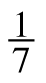x5 + 9

3. f(x) = 5(x + 3)(x − 2)(x + 7)

4. y = (x − 3)2(x + 1)(x3 + 1)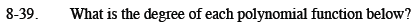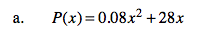Look for the highest-valued exponent.

2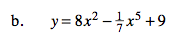See part (a).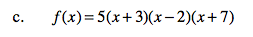If you multiplied the factors to write the function in standard form, what would be the highest power of x?

3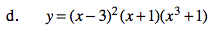See part (c).